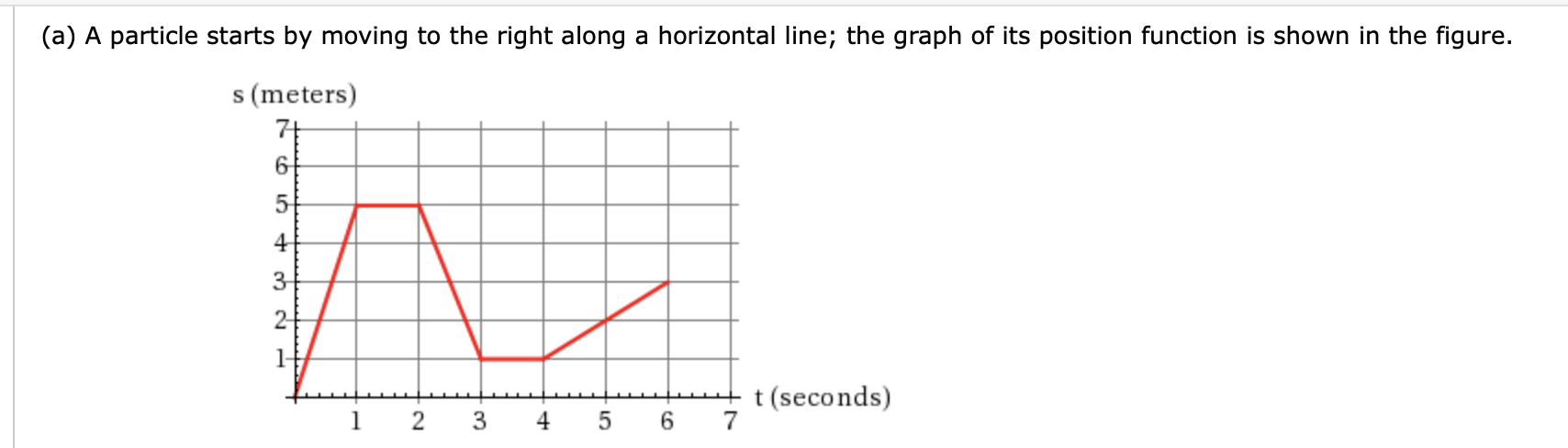# (a) A particle starts by moving to the right along a horizontal line; the graph of its position function is shown in the figure.s (meters)432-t(seconds)-NCO-LO

Question
27 views

Please draw a handwritten graph of the velocity function with data points.help_outlineImage Transcriptionclose(a) A particle starts by moving to the right along a horizontal line; the graph of its position function is shown in the figure. s (meters) 4 3 2- t(seconds) -N CO -LO fullscreen
check_circle

Step 1

We find rate of changes at every interval first.

From x=0 to x=1, i.e. from (0,0) to (1,5) rate of change= (5-0)/(1-0)=5

From x=1 to x=2, i.e. from (1,5) to (2,5) rate of change=(5-5)/(2-1)=0

From x=2 to x=3, i.e. from (...

### Want to see the full answer?

See Solution

#### Want to see this answer and more?

Solutions are written by subject experts who are available 24/7. Questions are typically answered within 1 hour.*

See Solution
*Response times may vary by subject and question.
Tagged in

### Calculus